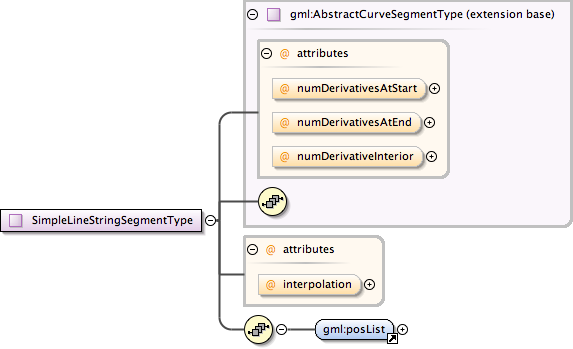### Showing:

 Annotations Attributes Diagrams Facets Properties Source Used by
Complex Type metobj:SimpleLineStringSegmentType
Namespace http://xml.fmi.fi/namespace/meteorology/conceptual-model/meteorological-objects/2009/11/09
Annotations
 ```A SimpleLineStringSegment is a curve segment that is defined by two or more coordinate tuples, with linear interpolation between them. Note: SimpleLineStringSegment implements GM_LineString of ISO 19107, but allows only one way of specifying the direct positions.```
DiagramType extension of gml:AbstractCurveSegmentType
Type hierarchy
Used by
 Element metobj:SimpleLineStringSegment
Model gml:posList
Children gml:posList
Attributes
QName Type Fixed Default Use Annotation
interpolation gml:CurveInterpolationType linear optional
 ```The attribute "interpolation" specifies the curve interpolation mechanism used for this segment. This mechanism uses the control points and control parameters to determine the position of this curve segment. For a SimpleLineStringSegment the interpolation is fixed as "linear".```
numDerivativeInterior integer 0 optional
 ```The attribute "numDerivativesInterior" specifies the type of continuity that is guaranteed interior to the curve. The default value of "0" means simple continuity, which is a mandatory minimum level of continuity. This level is referred to as "C 0 " in mathematical texts. A value of 1 means that the function and its first derivative are continuous at the appropriate end point: "C 1 " continuity. A value of "n" for any integer means the function and its first n derivatives are continuous: "C n " continuity. NOTE: Use of these values is only appropriate when the basic curve definition is an underdetermined system. For example, line string segments cannot support continuity above C 0 , since there is no spare control parameter to adjust the incoming angle at the end points of the segment. Spline functions on the other hand often have extra degrees of freedom on end segments that allow them to adjust the values of the derivatives to support C 1 or higher continuity.```
numDerivativesAtEnd integer 0 optional
 ```The attribute "numDerivativesAtEnd" specifies the type of continuity between this curve segment and its successor. If this is the last curve segment in the curve, one of these values, as appropriate, is ignored. The default value of "0" means simple continuity, which is a mandatory minimum level of continuity. This level is referred to as "C 0 " in mathematical texts. A value of 1 means that the function and its first derivative are continuous at the appropriate end point: "C 1 " continuity. A value of "n" for any integer means the function and its first n derivatives are continuous: "C n " continuity. NOTE: Use of these values is only appropriate when the basic curve definition is an underdetermined system. For example, line string segments cannot support continuity above C 0 , since there is no spare control parameter to adjust the incoming angle at the end points of the segment. Spline functions on the other hand often have extra degrees of freedom on end segments that allow them to adjust the values of the derivatives to support C 1 or higher continuity.```
numDerivativesAtStart integer 0 optional
 ```The attribute "numDerivativesAtStart" specifies the type of continuity between this curve segment and its predecessor. If this is the first curve segment in the curve, one of these values, as appropriate, is ignored. The default value of "0" means simple continuity, which is a mandatory minimum level of continuity. This level is referred to as "C 0 " in mathematical texts. A value of 1 means that the function and its first derivative are continuous at the appropriate end point: "C 1 " continuity. A value of "n" for any integer means the function and its first n derivatives are continuous: "C n " continuity. NOTE: Use of these values is only appropriate when the basic curve definition is an underdetermined system. For example, line string segments cannot support continuity above C 0 , since there is no spare control parameter to adjust the incoming angle at the end points of the segment. Spline functions on the other hand often have extra degrees of freedom on end segments that allow them to adjust the values of the derivatives to support C 1 or higher continuity.```
Source
 ``` A SimpleLineStringSegment is a curve segment that is defined by two or more coordinate tuples, with linear interpolation between them. Note: SimpleLineStringSegment implements GM_LineString of ISO 19107, but allows only one way of specifying the direct positions. Unlike in gml:LineStringSegmentType, positions can only be specified in one way: inlined using gml:posList. The number of direct positions in the list must be at least two. The attribute "interpolation" specifies the curve interpolation mechanism used for this segment. This mechanism uses the control points and control parameters to determine the position of this curve segment. For a SimpleLineStringSegment the interpolation is fixed as "linear". ```
Schema location http://xml.fmi.fi/schema/meteorology/conceptual-model/meteorological-objects/2009/11/09/metobjects-common-geometry.xsd
Attribute metobj:SimpleLineStringSegmentType / @interpolation
Namespace No namespace
Annotations
 ```The attribute "interpolation" specifies the curve interpolation mechanism used for this segment. This mechanism uses the control points and control parameters to determine the position of this curve segment. For a SimpleLineStringSegment the interpolation is fixed as "linear".```
Type gml:CurveInterpolationType
Properties
 fixed: linear
Facets
 enumeration linear enumeration geodesic enumeration circularArc3Points enumeration circularArc2PointWithBulge enumeration circularArcCenterPointWithRadius enumeration elliptical enumeration clothoid enumeration conic enumeration polynomialSpline enumeration cubicSpline enumeration rationalSpline
Used by
 Complex Type metobj:SimpleLineStringSegmentType
Source
 ``` The attribute "interpolation" specifies the curve interpolation mechanism used for this segment. This mechanism uses the control points and control parameters to determine the position of this curve segment. For a SimpleLineStringSegment the interpolation is fixed as "linear". ```
Schema location http://xml.fmi.fi/schema/meteorology/conceptual-model/meteorological-objects/2009/11/09/metobjects-common-geometry.xsd